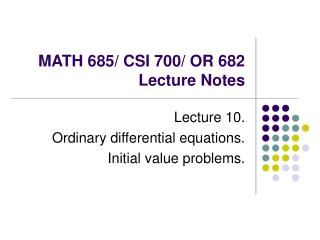DownloadDownload PresentationMATH 685/ CSI 700/ OR 682 Lecture Notes

MATH 685/ CSI 700/ OR 682 Lecture Notes

Download PresentationMATH 685/ CSI 700/ OR 682 Lecture Notes

- - - - - - - - - - - - - - - - - - - - - - - - - - - E N D - - - - - - - - - - - - - - - - - - - - - - - - - - -
Presentation Transcript

1. MATH 685/ CSI 700/ OR 682 Lecture Notes Lecture 10. Ordinary differential equations. Initial value problems.

2. Differential equations • Differential equations involve derivatives of unknown solution function • Ordinary differential equation (ODE): all derivatives are with respect to single independent variable, often representing time • Solution of differential equation is function in infinite-dimensional space of functions • Numerical solution of differential equations is based on finite-dimensional approximation • Differential equation is replaced by algebraic equation whose solution approximates that of given differential equation

3. Order of ODE • Order of ODE is determined by highest-order derivative of solution function appearing in ODE • ODE with higher-order derivatives can be transformed into equivalent first-order system • We will discuss numerical solution methods only for first-order ODEs • Most ODE software is designed to solve only first-order equations

4. Higher-order ODEs

5. Example: Newton’s second law u1 = solution y of the original equation of 2nd order u2 = velocity y’ Can solve this by methods for 1st order equations

6. ODEs

7. Initial value problems

8. Initial value problems

9. Example

10. Example (cont.)

11. Stability of solutions Solution of ODE is • Stable if solutions resulting from perturbations of initial value remain close to original solution • Asymptotically stable if solutions resulting from perturbations converge back to original solution • Unstable if solutions resulting from perturbations diverge away from original solution without bound

12. Example: stable solutions

13. Example: asymptotically stable solutions

14. Example: stability of solutions

15. Example: linear systems of ODEs

16. Stability of solutions

17. Stability of solutions

18. Numerical solution of ODEs

19. Numerical solution to ODEs

20. Euler’s method

21. Example

22. Example (cont.)

23. Example (cont.)

24. Example (cont.)

25. Numerical errors in ODE solution

26. Global and local error

27. Global vs. local error

28. Global vs. local error

29. Global vs. local error

30. Order of accuracy. Stability

31. Determining stability/accuracy

32. Example: Euler’s method

33. Example (cont.)

34. Example (cont.)

35. Example (cont.)

36. Example (cont.)

37. Stability in ODE, in general

38. Step size selection

39. Step size selection

40. Implicit methods

41. Implicit methods, cont.

42. Backward Euler method

43. Implicit methods

44. Backward Euler method

45. Backward Euler method

46. Unconditionally stable methods

47. Trapezoid method

48. Trapezoid method

49. Implicit methods

50. Stiff differential equations